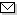Language:   Search:   ContactSearch and Browse the MSC 2000

Search:
Please note that in the second half of 2009, MSC2010 is deployed in the production of ZMATH and will become official standard in January 2010.

Enter a query and click »Search«...

49-XX Calculus of variations and optimal control; optimization [See also 34H05, 34K35, 65Kxx, 90Cxx, 93-XX] → ZMATH
49Jxx Existence theories → ZMATH
49J05 Free problems in one independent variable → ZMATH
49J10 Free problems in two or more independent variables → ZMATH
49J15 Optimal control problems involving ordinary differential equations → ZMATH
49J20 Optimal control problems involving partial differential equations → ZMATH
49J22 Optimal control problems involving integral equations → ZMATH
49J24 Optimal control problems involving differential inclusions [See also 34A60] → ZMATH
49J25 Optimal control problems involving equations with retarded arguments [See also 34K35] → ZMATH
49J30 Optimal solutions belonging to restricted classes (Lipschitz controls, bang-bang controls, etc.) → ZMATH
49J35 Minimax problems → ZMATHAbel prize 2010I. M. Gelfand 1913-2009MSC2010